# 6 Machine Learning Algorithms You Should Learn as a Newbie

2
1912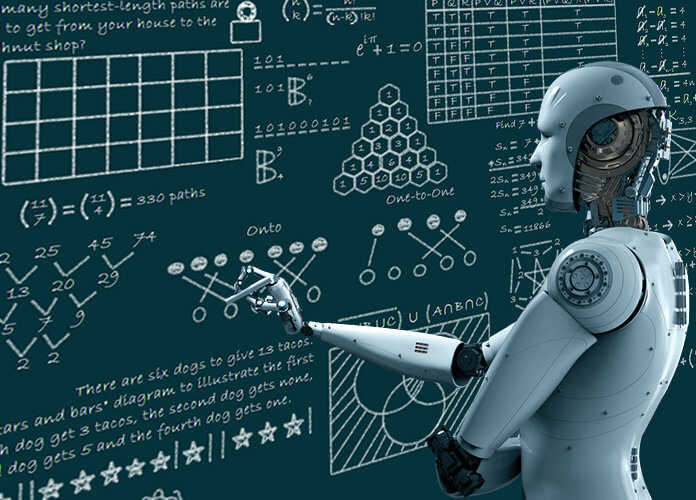In our present world, the definition of manual is changing since a lot of tasks are being automated. Machine Learning algorithms are helping computers play chess, perform surgeries, process data in a meaningful way, learn how to drive cars, thus, helping them to get smarter.

There is no doubt that machine learning along with artificial intelligence is gaining more popularity and becoming more accessible in past couple of years. These machine learning algorithms are mainly categorized into three different broad categories: Supervised, Unsupervised, and Reinforcement. Let us take a look at what they exactly mean.

## 3 Main Categories of Machine Learning Algorithms

### Supervised Algorithms

In this category, a certain data set is available as an input, commonly known as training data set. During this session, the model will adjust variables provided as input to map to the corresponding output.

### Unsupervised Algorithms

These algorithms are useful where the challenge is to discover the indirect relationships in a given data set. There is no one desired outcome.

### Reinforcement Algorithms

The working model of these algorithms is based on decisions. The algorithms in this category train themselves based on those decisions outcome, as per the success or the error. They learn from the feedback available.

Decision Trees

It uses a tree-like graph or model of decisions and their possible outcomes. To make a correct decision, the model describes a minimum number of questions to ask. It approaches a problem in a structured and a systematic way to find a solution that is logical. Mainly, the algorithm is used to solve classification problems.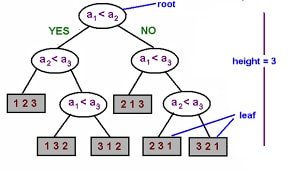For example, consider a population of people that use the decision tree algorithm to identify who will like to have a credit card. The age and employment status are the properties used. If a person’s age is over 25 and the person is employed, they will tend to have a credit card.

Logistic Regression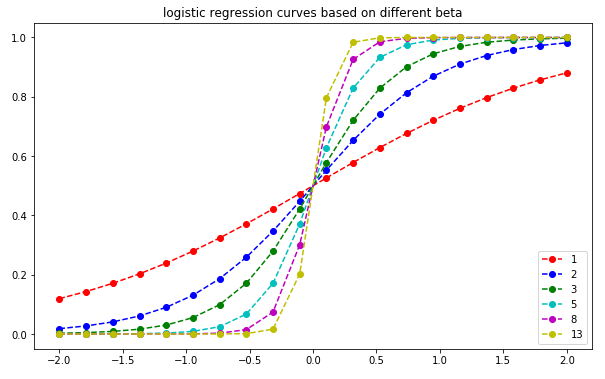Logistic Regression has a proper use case where a set of independent variables are available and the algorithm is used to estimate discrete values out of them. It helps in predicting the probability of an event by using a logistics function.

Some of the real world examples are predicting the revenues of a specific product in a category of its own and estimating the chances of an earthquake happening in a prone area on a particular day.

Linear Regression

This algorithm uses data points to find the best model for the available data set. It does this in terms of a line. A line is represented by the equation y = m * x + c and is known as the regression line, where y is the dependent variable, x is the independent variable and using the given data set, calculus is applied to find the values for m and c.

The relationship established between the dependent and the independent variable is the outcome in form of a line. A real world example of this algorithm will be the amount of crop yielding to rainfall where yield is the dependent variable and rainfall is the independent variable.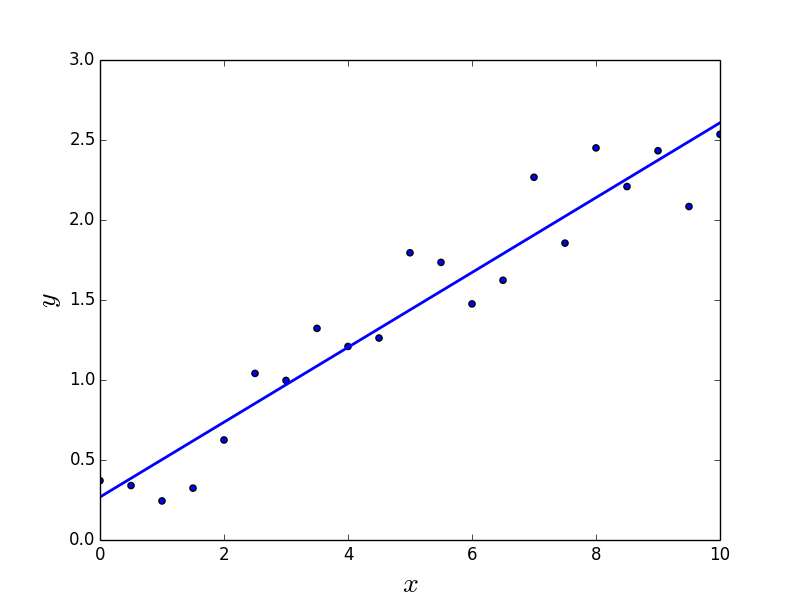Naive Bayes Algorithm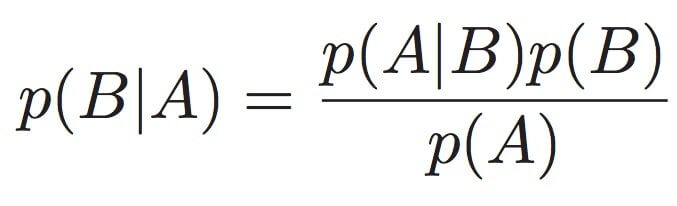Based on the Bayes Theorem in probability. It is a classification algorithm and is applied in only those use cases where the features or labels in a problem are independent of each other. This is the basic requirement of Bayes Theorem.

Some of the major use cases of this algorithm are applicable to real world problems such as labeling an email as spam, checking an article from the already defined categories such as politics, sports, etc. and is also used in face recognition software.

Random Forest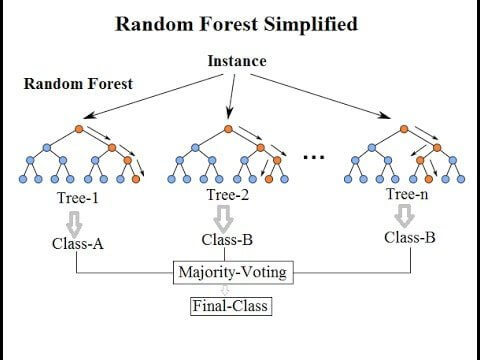Random Forest algorithm is a collection of decision trees in which each tree is trying to estimate a classification based on available attributes as instances and this is known as a vote. To reach an outcome, each vote from every tree is taken into consideration and the outcome is the most voted classification. In this way, it helps to determine the most important features of a data set.

A real-world example of using this algorithm is in pharmaceutical and medical. It can be used to identify the correct combination of components in medicine and to identify disease based on a patient’s medical records by analysis.

K-Means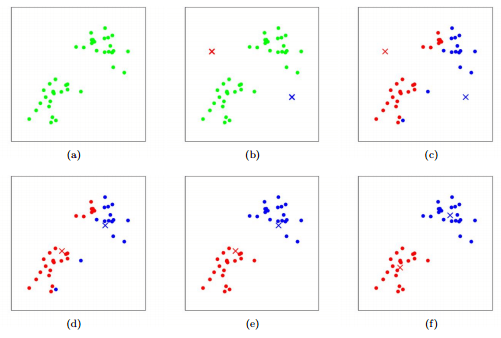This algorithm is used to provide a solution for a clustering problem. It falls under the category of unsupervised algorithms. It follows a dataset (multiple datasets) to form a number of clusters in a way such that all the data points within a cluster are homogeneous. These clusters are generated by the input to the algorithm which is the value of k.

It picks k number of points called centroids in each cluster. Each neighboring data point then forms a new cluster with the closest centroids. These new centroids are based on the existing number of clusters. This process is iterated over and over until each centroid is left with no ability to change.

These are just a few of the many different types of ML Algorithms that are currently on the market. However, if you are a newbie who wants to get into Machine Learning, then these 6 algorithms are a must for you to get started. So, strap on your boots and get started with these algorithms.

1.Lisa
•Tutor @ Eduonix